Next: Classification of the problems Up: Introduction Previous: Gaps and Trees

## Scoring Functions

In all our examples, the scoring function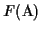of an MSA is similar to the sum-of-pairs measure , except that we do not add all scores of all pairwise alignments, but only the scores of the pairwise alignments in a circular order C(S) with respect to the associated evolutionary tree. Such an order can be determined without the knowledge of an evolutionary tree. For a detailed explanation see .

Definition 1.5   The score of the induced MSA-derived pairwise alignment
MPA(ai, aj) of two sequences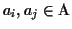is the score of the alignment of the two strings ai and aj, where any scoring function for pairwise alignments can be used. Opposing gaps may be removed.

Usually the optimal score for pairwise alignments is determined via standard dynamic programming [18,19]. An affine gap cost is used according to the formula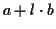, where a is a fixed gap cost, l is the length of the gap and b is the incremental cost . The circular sum score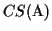of an MSA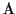is the sum of MPA scores in circular order C divided by two:

Definition 1.6   The scoreof an MSAis defined as: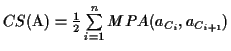where Cn+1 = C1, and C is a circular order with respect to the evolutionary tree of.

Whenever we use the term score of an MSA, we mean the CS score. The term "best possible score" of an MSA refers to the CS score using the optimal scores of the pairwise alignments instead of the MPA scores. For a better explanation see . In the following we classify the major problems that can arise when MSAs are built. We then present an algorithm that is derived from biological observation to improve MSAs. Then we show how to reconstruct an evolutionary tree from the gaps. Furthermore, we show how the solution of a vertex cover problem can be used for resolving conflicts that can arise when constructing trees from gaps.Next: Classification of the problems Up: Introduction Previous: Gaps and Trees
Chantal Korostensky
1999-07-14